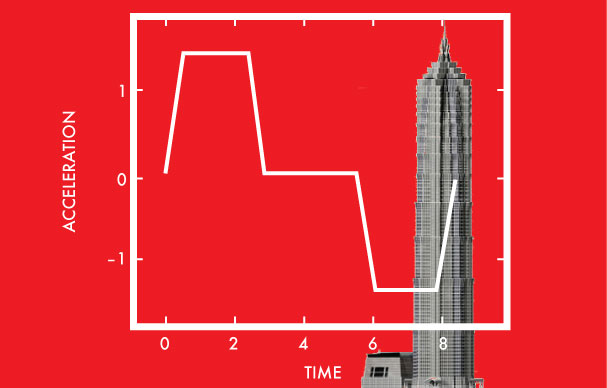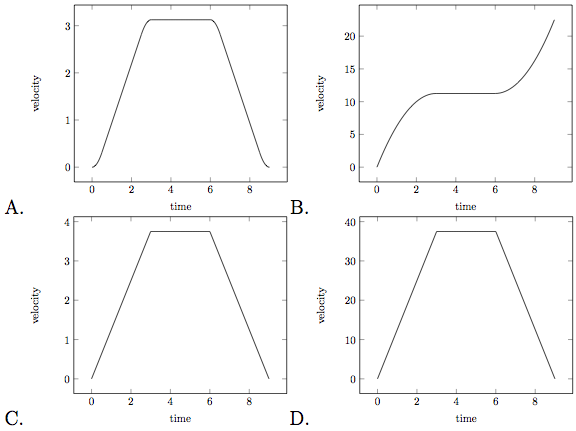Calculus

# Finding Velocity given AccelerationThe Shanghai Tower is equipped with the world fastest elevator, which can travel at $18\, m/s$!.

As the elevator takes time to accelerate, and decelerate, the acceleration graph can be modeled as indicated in the above diagram.

Which of the following is the best graph to illustrate the velocity of the elevator?A rocket starts at rest with acceleration at time $t$ given by $a(t)=\left(6t^2+10t\right) \text{ m/s}^2.$ What is its velocity (in $\text{ m/s}$) at $t=10$ seconds?

The acceleration of a particle traveling in a straight line is given by the equation $a(t)=2t.$ If the velocity at $t=1$ is $v=4$ and the distance from the starting point at $t=1$ is $s=15$, what is the distance from the starting point at $t=2?$

The acceleration of an object in motion is given by the vector $\overrightarrow{a}(t)=(4t,e^t).$ If the object's initial velocity is $\overrightarrow{v}(0)=(8,0),$ which of the following is the velocity vector as a function of time $t?$

The position of a particle at time $t$ is given by vector $$ and its acceleration is given by $\mathbf{a}=<-12\cos 2t, -7\sin t>$ for $-\frac{\pi}{2} \leq t \leq \frac{\pi}{2}$. For $t=0,$ the position vector of the particle is $<3,0>$ with $\displaystyle \frac{dx}{dt}=0$ and $\displaystyle \frac{dy}{dx}=7.$ Find the position vector $$.

×